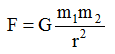Ex﻿

# 4. Gravitation

#### Newton's Law Of Gravitation

According to newton, "Any two bodies in universe attract each other with a force directly proportional to the product of their masses and inversely proportional to the square of the distance between them."
Mathematical Expression
Lat A and B be two particle of mass m1 and m2 respectively. Let the distance AB = r.
By the law of gravitational, the particle A attracts the particle B with a force F such that.F ∝ m1m2 (for a given pair of particles)
F ∝(for a given separation between the particles)
SoHere G is a constant known as the universal constant of gravitation. G = 6.67 × 10–11 Nm2/kg2
G is independent of the masses of the bodies and the distance between them.
Newton's law of gravitation is applicable for everybody in the universe.
Note : The force between any two bodies in the universe is called the force of gravitation while the force with which earth attracts a body is called the force of gravity.

Some Scientific Phenomenon Based on Gravitational Force :
The gravitational force between the sun and the earth keeps the earth moving around the sun.
The gravitational force b/w the earth and the moon keeps the moon moving around the earth.
Existence of our solar system is due to gravitational force.
Gravitation force of the sun and the moon on the earth's water surface is responsible for the tides in sea.
Atmosphere above the earth is held due to gravitational force of the earth.
Gravitational force between the sun and planet keeps the planet moving around the sun.
Gravitational force is responsible for providing the centripetal force required by the planets.
The attractive force of the earth is responsible for providing the centripetal force required by moon.

If you want to give information about online courses to other students, then share it with more and more on Facebook, Twitter, Google Plus. The more the shares will be, the more students will benefit. The share buttons are given below for your convenience.
×

#### NTSE Physics (Class X)

• Electricity
• Magnetic Effect of Curent
• Light Reflection
• Light Refraction
• Human Eye & Colourful World
• Source of Energy
• Motion

#### NTSE Physics (Class IX)

• Force & laws of motion
• Work and Energy
• Gravitation
• Sound

SHOW CHAPTERS